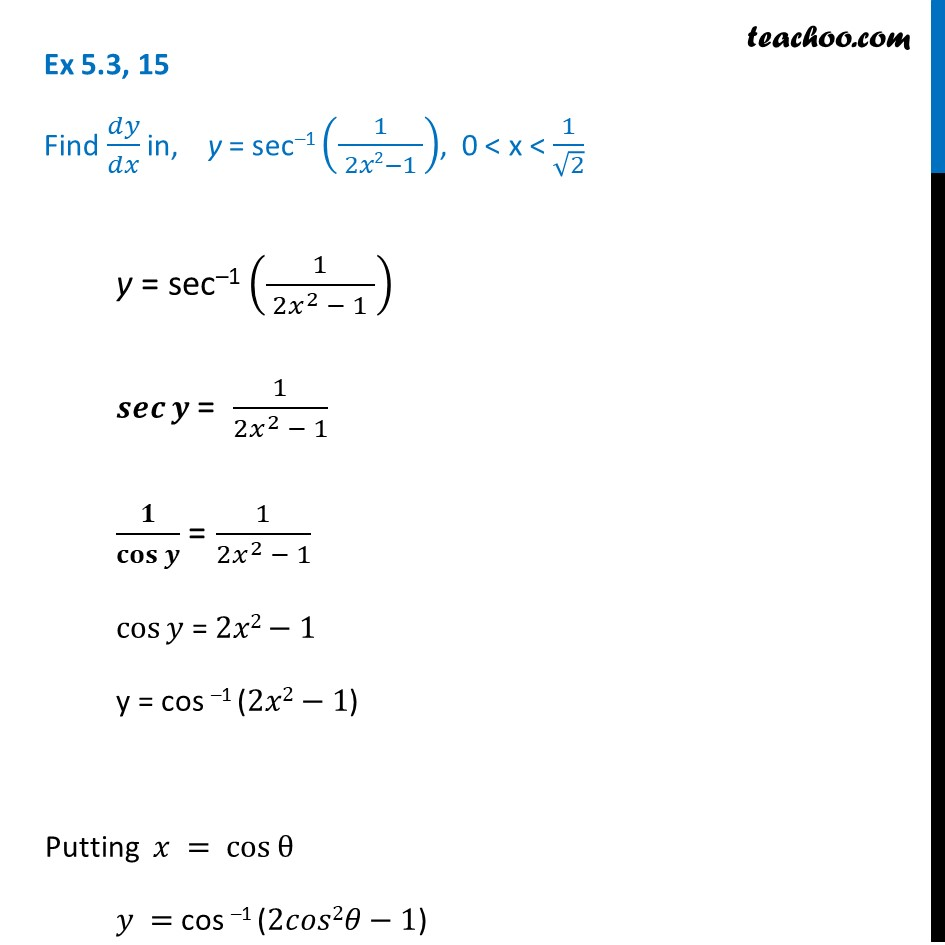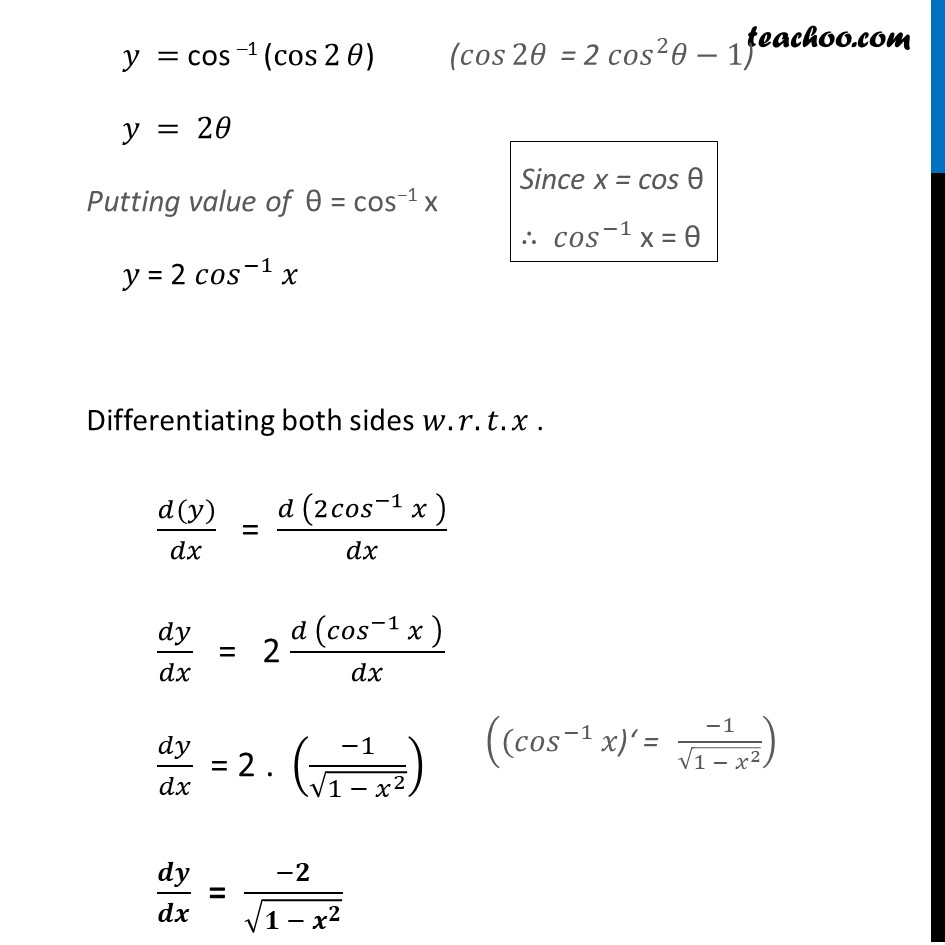Finding derivative of Inverse trigonometric functions

Chapter 5 Class 12 Continuity and Differentiability
Concept wiseIntroducing your new favourite teacher - Teachoo Black, at only ₹83 per month

### Transcript

Ex 5.3, 15 Find 𝑑𝑦/𝑑𝑥 in, y = sec–1 (1/( 2𝑥2−1 )), 0 < x < 1/√2 y = sec–1 (1/( 2𝑥^2 − 1 )) 𝒔𝒆𝒄⁡𝒚 = 1/(2𝑥^2 − 1) 𝟏/𝐜𝐨𝐬⁡𝒚 = 1/(2𝑥^2 − 1) cos⁡𝑦 = 2𝑥2−1 y = cos –1 (2𝑥2−1) Putting 𝑥 = cos⁡θ 𝑦 = cos –1 (2𝑐𝑜𝑠2𝜃−1) 𝑦 = cos –1 (cos⁡2 𝜃) 𝑦 = 2𝜃 Putting value of θ = cos−1 x 𝑦 = 2 〖𝑐𝑜𝑠〗^(−1) 𝑥 Differentiating both sides 𝑤.𝑟.𝑡.𝑥 . (𝑑(𝑦))/𝑑𝑥 = (𝑑 (2〖𝑐𝑜𝑠〗^(−1) 𝑥" " ))/𝑑𝑥 𝑑𝑦/𝑑𝑥 = 2 (𝑑 (〖𝑐𝑜𝑠〗^(−1) 𝑥" " ))/𝑑𝑥 𝑑𝑦/𝑑𝑥 = 2 . ((−1)/√(1 − 𝑥^2 )) 𝒅𝒚/𝒅𝒙 = (−𝟐)/√(𝟏 − 𝒙^𝟐 ) (𝑐𝑜𝑠⁡2𝜃 " = 2 " 〖𝑐𝑜𝑠〗^2 𝜃−1) Since x = cos θ ∴ 〖𝑐𝑜𝑠〗^(−1) x = θ ((〖𝑐𝑜𝑠〗^(−1) 𝑥")‘ = " (−1)/√(1 − 𝑥^2 ))